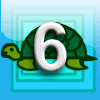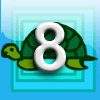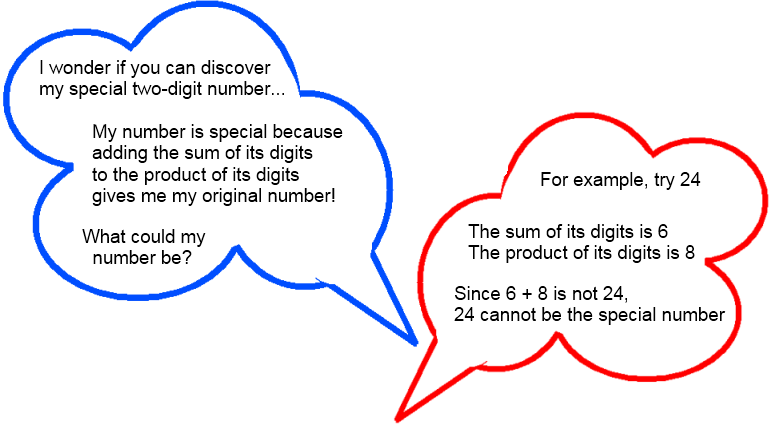#### You may also likeThink of a number and follow the machine's instructions... I know what your number is! Can you explain how I know?### First Forward Into Logo 6: Variables and Procedures

Learn to write procedures and build them into Logo programs. Learn to use variables.### First Forward Into Logo 8: More about Variables

Write a Logo program, putting in variables, and see the effect when you change the variables.

# Special Numbers

##### Age 11 to 14Challenge Level

One day our teacher asked us a puzzling question:Can you find a special number?
Can you find more than one?
Can you find them all?

One way to convince yourself that you've found all the special numbers is to use a little algebra...
Any two-digit number can be represented algebraically as $10a + b$.
$a$ is the tens digit and $b$ is the units digit.

Write down the algebraic expressions for the sum and the product of the digits.
Now write an equation that all special numbers will satisfy. See the hint if you're not sure how to do this.
Solve the equation, and use it to convince yourself that you have found all the special numbers.

There are other sorts of special two-digit numbers...

• I add twice the tens digit to the units digit, then add this to the product of the digits. I get back to my original number.

• I add three times the tens digit to the units digit, then add this to the product of the digits. I get back to my original number.

• I add four times... or five times... or...
Can you use algebra to help you to find these special numbers?